# Not Equal To in Excel

In Excel, <> means not equal to. The <> operator in Excel checks if two values are not equal to each other. Let's take a look at a few examples.

1. The formula in cell C1 below returns TRUE because the text value in cell A1 is not equal to the text value in cell B1.2. The formula in cell C1 below returns FALSE because the value in cell A1 is equal to the value in cell B1.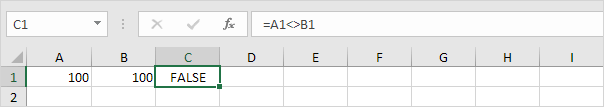3. The IF function below calculates the progress between a start and end value if the end value is not equal to an empty string (two double quotes with nothing in between), else it displays an empty string (see row 5).4. The COUNTIF function below counts the number of cells in the range A1:A5 that are not equal to "red".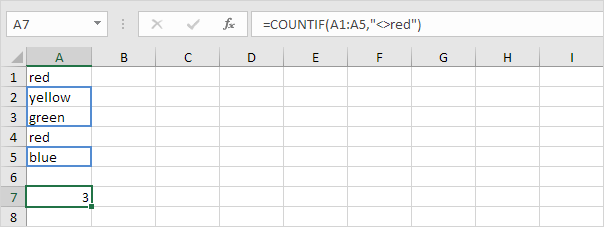5. The COUNTIF function below produces the exact same result. The & operator joins the 'not equal to' operator and the text value in cell C1.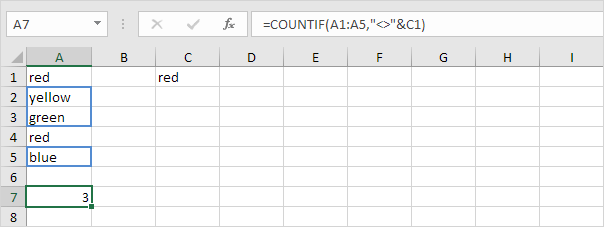6. The COUNTIFS function below counts the number of cells in the range A1:A5 that are not equal to "red" and not equal to "blue".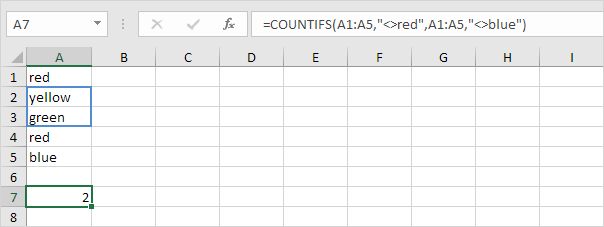Explanation: the COUNTIFS function in Excel counts cells based on two or more criteria. This COUNTIFS function has 2 range/criteria pairs.

7. The AVERAGEIF function below calculates the average of the values in the range A1:A5 that are not equal to 0.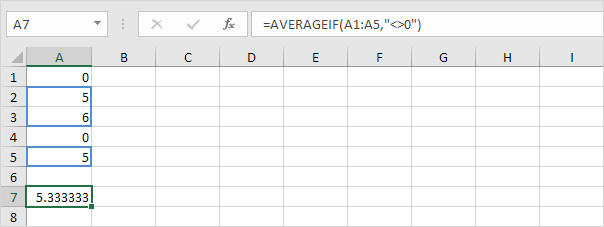Note: in other words, the AVERAGEIF function above calculates the average excluding zeros.

Go to Next Chapter: Logical Functions